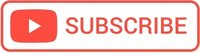# Using functions in Python

A function is a reusable block of code that is used to perform a single action and is executed only when called.
You can pass data, known as parameters, into a function.

## Creating a Function

To start the definition of a function, use the def keyword.

## Return value from a function

The return keyword is used when You want to return a value.

The return statement ends the execution of the function call and returns the result.

def hello():
return "Hello Webpedia"
print(hello())

//Hello Webpedia


or

def hello():
return "Hello"
print(hello(), "Webpedia")

//Hello Webpedia


The return keyword can be anywhere inside a function body, although it is the last line of the function.

A function can have multiple return statements.

### Functions that don’t return

What would happen if we didn’t include the return keyword in our function?
The result of calling them is the None value. print() or help() are example of functions that don’t return anything.

### Docstrings

The docstring is a triple-quoted string (which may span multiple lines) that comes immediately after the header of a function.

When we call help() on a function, it shows the docstring.

### Default arguments

def greeting(name='Webpedia'):
print("Hello,", name)


Also, You can supply functions as arguments to other functions.

def greeting(name='Webpedia'):
print("Hello,", name)
def call(fn, arg):
"""Call fn on arg"""
return fn(arg)

call(greeting, 'again')

//Hello, again


Functions that operate on other functions are called “Higher order functions.”

### pass keyword

“pass” is a keyword that does literally nothing. It is used as a placeholder, because after we begin a code block, Python requires at least one line of code.

def greeting():
pass


## Global vs. local variables in functions

#Global scope
x = "abc"

def myFunction():
#Local scope
x = "var with local scope"
print(x)

myFunction()

#var with local scope

#Global scope
x = "abc"

def myFunction():
#Local scope
x = "var with local scope"
print(x)

print(x)
#abc


If you use the global keyword to a variable inside a function, the purpose of the variable becomes global:

x = "abc"

def myFunction():
global x
x = "var with local scope"
print(x)

myFunction()
#var with local scope

print(x)
#var with local scope

<h2>Exercises and examples</h2>

def hello(lang):
if lang == 'en':
return "Hello Webpedia"
elif lang == 'fr':
return "Bonjour Webpedia"
else:
return "Hi Webpedia"

print(hello('en'))
//Hello Webpedia

print(hello('xyz'))
//Hi Webpedia


A function can have more than one parameter.

def sum(a,b):
c = a + b
return c
sum = sum(1,4)
print(sum)

//5


## Python Function order of parsing arguments

As seen in the example below, You don't have to call the function with the arguments in a particular order, if you supply the names along with the values:

def power(num,x=1):
result = 1
for i in range(x):
result = result * num
return result

print(power(5))
#5

print(power(5,2))
#25

print(power(x=2,num=5))
#25


## Passing multiple arguments to a function in Python

*args and **kwargs allows You to pass multiple arguments or keyword arguments to a function.

The star character (*) means we can pass a variable number of arguments.

def multi_sum(*args):
result = 0
for x in args:
result += x
return result

print(multi_sum(5,5,1))


Important!! The variable argument list always has to be the last parameter!

## Python functions are first-class values

### Unlike other programming languages, Python does not have a switch or case statement.

In Python, functions behave like any other object, such as an int, float, string, or list. That means you can use functions as arguments to other functions, or store functions as dictionary values, or return a function from another function, etc

Following this approach, You can easily replace the switch statement using a dictionary.

## Question: Why is the code below often added to a Python program file?

if __name__ == "__main__":
main()

It executes the main() function only if this file is executed as the main program.

#### Hello there!

I hope you find this post useful!

I'm Mihai, a programmer and online marketing specialist, very passionate about everything that means online marketing, focused on eCommerce.

If you have a collaboration proposal or need helps with your projects feel free to contact me. I will always be glad to help you!